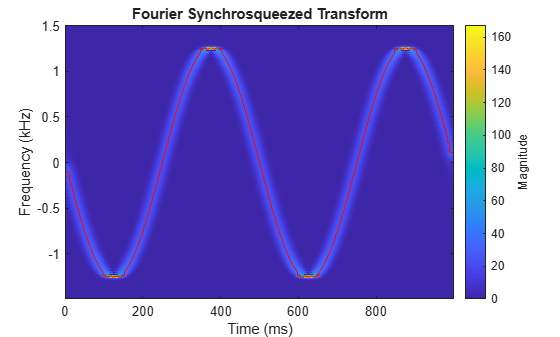Main Content

# Instantaneous Frequency of Complex Chirp

This example shows how to compute the instantaneous frequency of a signal using the Fourier synchrosqueezed transform.

Generate a chirp with sinusoidally varying frequency content. The signal is embedded in white Gaussian noise and sampled at 3 kHz for 1 second.

```fs = 3000; t = 0:1/fs:1-1/fs; x = exp(2j*pi*100*cos(2*pi*2*t)) + randn(size(t))/100;```

Compute and plot the Fourier synchrosqueezed transform of the signal. Display the time on the x-axis and the frequency on the y-axis.

`fsst(x,fs,'yaxis')`Find the instantaneous frequency of the signal by extracting the maximum-energy time-frequency ridge of the Fourier Synchrosqueezed transform.

```[sst,f,tfs] = fsst(x,fs); fridge = tfridge(sst,f);```

Overlay the ridge on the transform plot. Convert time to milliseconds and frequency to kHz.

```hold on plot(t*1000,fridge/1000,'r') hold off```For a real signal, you can find the instantaneous frequency more easily using the `instfreq` function. For example, display the instantaneous frequency of the real part of the complex chirp by computing the analytic signal and differentiating its phase.

```ax = real(x); instfreq(ax,fs,'Method','hilbert')```## Support

#### Deep Learning for Signal Processing with MATLAB

Download white paper